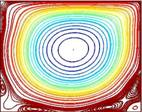Theoretical and Computational Aspects of the Streamfunction Form of a Ladyzhenskaya Model for Incompressible Viscous Flow Dr. Faisal A. Fairag, Assistant Professor, Math. Sciences   ( PI)

ABSTRACT

In this research project the streamfunction form of Ladyzhenskaya model is to be studied from both the theoretical and the computational point of views. In this research, an error analysis for finite element method of the proposed problem will be studied. In addition, the study will require writing a finite element computer code, and testing it on problems which have a known solution. An investigation of some parameters of the problem will be done in order to stabilize the code. The code will then be used to solve different physical problems such as the driven cavity problem. The code will also be validated through comparison with several published works. It is expected that this research will be significant to applied mathematicians and engineers working in the area of computational fluid dynamics and turbulent flows. It will open a new horizon for researchers in the area for further researches. Moreover, the developed code can be used to solve many physical problems. Upon the completion of this research, the expected outcomes are a MATLAB and FORTRAN codes in addition to the research technical report.

MATLAB & FORTRAN CODES